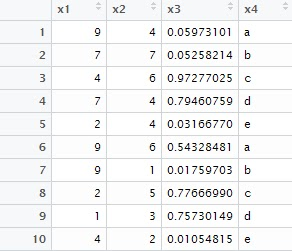# R Which Function Explained

This tutorial explains the usage of WHICH function in R and how it works with examples.

In R, the which() function gives you the position of elements of a logical vector that are TRUE. It can be a row number or column number or position in a vector.

Examples

1. What is the position of alphabet 'z' in a-z letters.

which(letters=="z")

It returns 26 as 'z' is positioned at 26th place.

Create a sample data frame

The following program creates a data frame consisting four variables. This data frame would be used in the subsequent examples.
ls = data.frame( x1 = ceiling(runif(10)*10),
x2 = ceiling(runif(10)*10),
x3 = runif(10),
x4= rep(letters[1:5],2))Sample Data Frame

2. Column number of variable "x4" in ls data set
i=which(names(ls)== "x4")
It returns 4 as x4 is placed at 4th column. It works like this -
names(ls) == "x4"  returns FALSE FALSE FALSE  TRUE. Then WHICH function tells R to calculate the position of TRUE.

3. Row number in which maximum value of variable "x1" exists
which(ls\$x1 == max(ls\$x1))
In this case, it gives you the row / observation number wherein the max value of x1 is stored.

4. Row number in which multiple conditions hold true
which(ls\$x1 == 7 & ls\$x2 == 4)
In this case, we are checking multiple conditions and figuring out the row number wherein conditions met.

5. Number of cases in which variable x1 is equal to variable x2
length(which(ls\$x1 == ls\$x2))
Let's break out in multiple steps -

1. which(ls\$x1 == ls\$x2) returns the position of all rows where these two variables are equal.
2. length() function calculates the length of the returned values of step 1.

6. Which value is common in both the variables
ls[which(ls\$x1 == ls\$x2),"x1"]
7. Extract names of all the numeric variables
check = which(sapply(ls, is.numeric))
colnames(ls)[check]
Let's run it step by step -

1. sapply(ls, is.numeric) returns TRUE  TRUE  TRUE FALSE. It's TRUE where variable is number else FALSE.

2. which(sapply(ls, is.numeric)) returns 1 2 3. Adding WHICH function returns the position in logical vectors.

3. colnames(ls)[check] returns x1 x2 and x3.Related PostsDeepanshu founded ListenData with a simple objective - Make analytics easy to understand and follow. He has over 8 years of experience in data science. During his tenure, he has worked with global clients in various domains like Banking, Insurance, Telecom and Human Resource.

3 Responses to "R Which Function Explained"
1.Hello,

I think that question 5 could be answered in a more concise way.
sum(ls\$x1 == ls\$x2)

What do you think of my answer?

Best regards
Cédric Guilmin

1.In R, there are multiple ways to accomplish the same task. I was just explaining the usage of 'WHICH' function. Yes, the question 5 should be answered with sum(ls\$x1 == ls\$x2) as it is more efficient.

Check out the following analysis -

ls = data.frame( x1 = ceiling(runif(10000000)*10),
x2 = ceiling(runif(10000000)*10))

start.time <- Sys.time()
length(which(ls\$x1 == ls\$x2))
end.time <- Sys.time()
time.taken <- end.time - start.time
time.taken

start.time <- Sys.time()
sum(ls\$x1 == ls\$x2)
end.time <- Sys.time()
time.taken <- end.time - start.time
time.taken

2.Next → ← Prev
Love this Post? Spread the Word!
Share